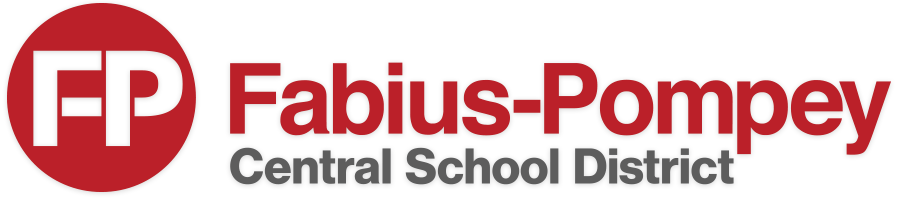Mr. Gilbert's Algebra Curriculum
The following list of topics shows the topics we will cover in Algebra this year, separated by unit, along with a general time frame.
KEY:

Red - Unit has been completed
Green - Unit is in progress
Black - Unit is still to come

Unit 1 - Numbers, Expressions and Equations (September - October)

• Rational and Irrational numbers
• Polynomial arithmetic
• Solving linear equations and inequalities
• Creating linear equations and inequalities
• Rearranging formulas
• Systems of linear equations
Unit 2 - Functions (October - November)
• Definition of function, domain and range
• Types of functions (linear, exponential, quadratic, square root, cube root, absolute value, piecewise, step)
• Function notation
• Average rate of change
• Sequences
• Linear vs Exponential functions
• Writing linear and exponential functions
• Interpreting linear and exponential functions
Unit 3 - Graphs, part 1 (November - December)
• Review y = mx + b
• Solving equations by graphing
• Graphing linear inequalities
• Features of graphs
• Average rate of change from a graph
Unit 4 - Graphs, part 2 (January)
• Graphing all types of functions
• Intercepts, maxima, minima
• Comparing functions
• Function transformations
• Constructing functions from a graph
• Graphs from verbal descriptions
Unit 5 - Quadratic Expressions and Functions (February)
• Factoring
• Finding zeros
• Completing the square to reveal vertex
Unit 6 - Solving Quadratic Equations (March)
• Solving quadratics with various methods (square roots, factoring, complete the square, quadratic formula)
• Pythagorean Theorem review
Unit 7 - Statistics (April)
• Displaying data
• Measures of center and variation
• Two-way tables
• Scatter plots
• Regression
• Correlation coefficient
• Residuals
Unit 8 - Regents Exam Review
• Topics TBD
DepartmentsAddress: 1211 Mill Street | Fabius, NY 13063
Phone: (315)683-5301